Science, Maths & Technology

### Become an OU student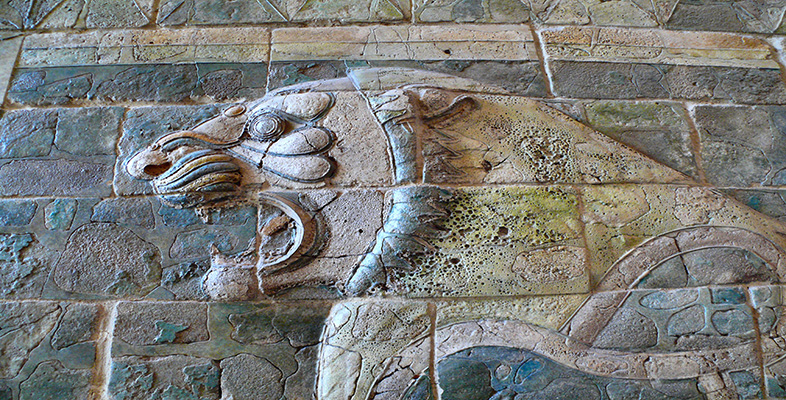Babylonian mathematics

Start this free course now. Just create an account and sign in. Enrol and complete the course for a free statement of participation or digital badge if available.

# 1.4 A remarkable numeration system

The Babylonian numeral system was described in Section 3 as ‘remarkable’. It is worth spelling out the reasons for this judgement. Although what we notice first is that it was a place-value system (see Box 1), what is perhaps more striking is the coupling of this feature with a ‘floating sexagesimal point’; that is, the lack of any indication about the absolute value of the number. This makes life hard for us in reading the tablets initially, but seems to have given the Babylonians unprecedented flexibility in calculations, because, among other things, there was no symbolic distinction between ‘whole numbers' and ‘fractions’.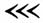could be 30, or 1800 (=30×60), or ½ (=30×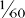), or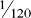(=30×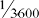), and so on. This approach completely sidesteps the relatively cumbersome Egyptian technique of handling fractional parts, and, together with the use of multiplication tables, leads arguably to computations even smoother than our own (at least before pocket calculators). We presume that, in any case where the absolute value of the number was significant, this would be clear to the scribe from the context. Also he would have needed to have kept his wits about him in doing addition or subtraction, where the places need to be lined up correctly. This system was used consistently only within mathematics, as far as we know. In dating, weights and measures, economic records and the like, there seems to have been a wide mixture of units with many local variations. (You saw an example earlier, in the ma-na to gin to se ratios of our first problem.)

It follows that there is a translation problem even in the task of finding equivalents in our system for what the Babylonian scribe wrote down. A helpful notation has been devised by Otto Neugebauer. He represents the value within each sexagesimal place in our numerals, separating the places by commas, (So the entries in the first column of the Larsa tablet would be transcribed as 40,1 41,40 43,21 and so on.) This leaves unspecified, just as the scribe did, the absolute value of a number. However, if we have reason to believe that we know where the ‘integer part’ of the number ends and the ‘fractional part’ begins, then a semi-colon is used to separate them. So, for instance, 1,10;30 would represent 60+10+30/60, which is 70½, or 70.5 in decimal fractions.

Now let us try this notation, and see more of the flexibility of Babylonian calculations. Below is an array of numbers from a different tablet, transcribed in Neugebauer's notation.

 2 30 16 3,45 45 1,20 3 20 18 3,20 4X 1,15 4 15 20 3 50 1,12 5 12 24 2,30 54 1,6,40 6 10 25 2,24 1 8 7,30 27 2,13,20 1.4 56,15 9 6,40 30 2 1,12 50 10 6 32 1,52,30 1,15 48 12 5 36 1,40 1,20 45 5 4 40 1,30 1,21 44,26,40

## Question 3

1. What do you think the table is about? (Hint: Try multiplying the paired numbers together and see if a pattern emerges.)

2. Observe that in the left-hand columns, certain numbers such as 7, 11, 13, etc. do not appear. Which ones are missing? Can you suggest a reason for this?

### Discussion

1. Let us take line 6, and multiply 7,30 by 8. Now, depending on whether we take 7,30 to be 7;30 (=7½), or 0;7,30 (i.e. 7/60 + 30/3600, =450/3600, =0.125 in decimal fractions), or 7,30;0 (= 450), when we multiply by 8 we get 60, or 1, or 3600 respectively. But these are all represented by the same cuneiform symbol,, and so it does not matter which we choose. You can reach an identical conclusion by looking at any other pair. In short, the table shows numbers which multiply together to give 1 (say), so we could call it a reciprocal table. The numbers in the right-hand columns are, in effect, 1 divided by the left-hand numbers (and vice versa—notice, for instance ‘45 1,20’ at the top right, and then ‘1,20 45’ lower down the same column).

2. If you try to divide 7, 11 or 13 into 60, and to express the result in sexagesimal fractions, you will find that it does not work exactly; the process is never-ending. More generally, notice that the only numbers appearing in the table are ones which are the result of multiplying together 2s, 3s and 5s in various combinations, and that 2, 3 and 5 are the only prime factors of 60 (=2×2×3×5). Thus 50 (=2×5×5) is in the table, but 21 (=3 × 7) is not. It is an arithmetical fact that only numbers whose prime factors are 2, 3 and 5 have reciprocals which can be expressed as finite (i.e. terminating) sexagesimal fractions. Such numbers we will call, following Neugebauer, regular numbers.

There are a couple of further points to make at this stage. There is some debate among historians about whether this is to be interpreted as a table of reciprocals, as suggested above, or whether its function was as a conversion table of fractional parts into their sexagesimal equivalent. (So 2 would stand for the second part, 3 the third part, and so on down to 1,21 as the eighty-first part, in something like the Egyptian mode.) In this interpretation, the columns would be not of numbers related reciprocally, but of the same number expressed in two different ways, as a unit fraction and as a sexagesimal fraction. This is an attractive idea, illustrating once again that it is harder than we should like to identify definitively even simple-looking tables. Fortunately, for our purposes, we do not need to resolve this point, so we shall continue to refer to the table as a reciprocal table.

Secondly, the Babylonians were quite able to divide by numbers other than regular ones, and approximated the results to three or four sexagesimal places. There are reciprocal tables for complete sequences of numbers (i.e. containing both regular and non-regular ones) from the period. Any division problem, therefore, could be converted into an equivalent multiplication one by using such tables. In order to divide by a number, you multiply by its reciprocal. If the number you are trying to divide by is regular, then the answer will be exact; otherwise, it will be approximate, (Note that the Babylonians had more regular numbers in the above sense than we have—any number with prime factors other than 2 and 5 lands us with an unending decimal fraction: e.g. ⅓=0.333… .)

We have now seen how the Babylonian numerals work, and also two examples of tables, one of squares and one of reciprocals, which give an idea of the level, spirit and flexibility of Babylonian computations. We did not try to ascertain how the results on the tables were arrived at, as that seemed either obvious or not very interesting. But thus equipped, we are ready to tackle a table whose method of construction does turn out to be rather interesting.